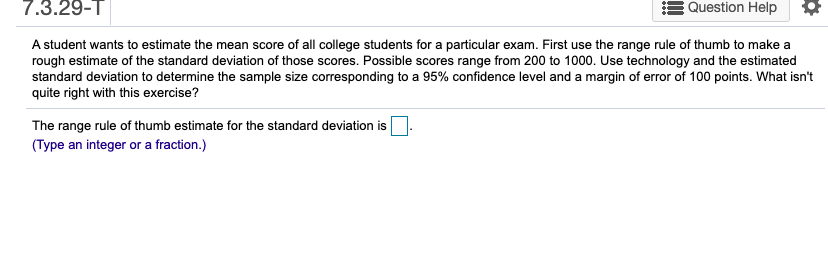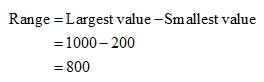# 7.3.29-1|Question HelpA student wants to estimate the mean score of all college students for a particular exam. First use the range rule of thumb to make arough estimate of the standard deviation of those scores. Possible scores range from 200 to 1000. Use technology and the estimatedstandard deviation to determine the sample size corresponding to a 95% confidence level and a margin of error of 100 points. What isn'tquite right with this exercise?The range rule of thumb estimate for the standard deviation is N(Type an integer or a fraction.)

Question
125 viewshelp_outlineImage Transcriptionclose7.3.29-1| Question Help A student wants to estimate the mean score of all college students for a particular exam. First use the range rule of thumb to make a rough estimate of the standard deviation of those scores. Possible scores range from 200 to 1000. Use technology and the estimated standard deviation to determine the sample size corresponding to a 95% confidence level and a margin of error of 100 points. What isn't quite right with this exercise? The range rule of thumb estimate for the standard deviation is N (Type an integer or a fraction.) fullscreen
check_circle

Step 1

Solution:

From the given information, the possible scores range from 200 to 1000.

Here, the largest value is 1000 and smallest value is 200....

### Want to see the full answer?

See Solution

#### Want to see this answer and more?

Solutions are written by subject experts who are available 24/7. Questions are typically answered within 1 hour.*

See Solution
*Response times may vary by subject and question.
Tagged in